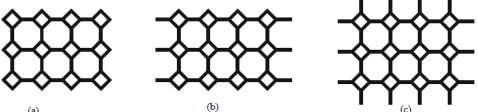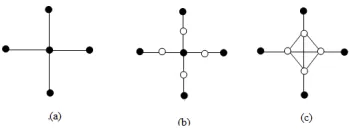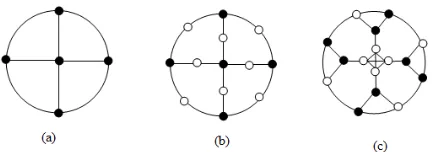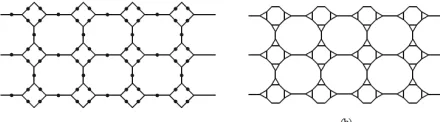# On the F-index and F-coindex of the line graphs of the subdivision graphs

N/A
N/A
Protected

Share "On the F-index and F-coindex of the line graphs of the subdivision graphs"

Copied!
7
0
0

Full text

(1)

https://doi.org/10.26637/MJM0602/0010

## the subdivision graphs

1

2

### *

Abstract

The aim of this work is to investigate the F-index and F-coindex of the line graphs of the cycle graphs, star graphs, tadpole graphs, wheel graphs and ladder graphs using the subdivision concepts. F-index of the line graph of subdivision graph of square grid graph, 2D-lattice, nanotube and nanotorus ofTUC4C8[p,q]are also investigated here.

Keywords

Topological index, F-index, F-coindex, line graphs, subdivision graphs, cycle graph, star graph, square grid graph, tadpole graphs, wheel graphs, ladder graphs, 2D-lattice ofTUC4C8[p,q], nanotube ofTUC4C8[p,q], nanotorus of TUC4C8[p,q].

AMS Subject Classification

05C35, 05C07, 05C40.

1,2Department of Mathematics, Aliah University, Kolkata -700 156, India.

*Corresponding author:2nayeemsma@gmail.com;1aminruhul80@gmail.com

Article History: Received09August2017; Accepted06March2018 c2018 MJM.

### Contents

1 Introduction. . . 362

2 F-index and F-coindex of some graphs using subdi-vision concept. . . .363

3 F-index of some nanostructures. . . 366

4 Conclusion. . . 367

References. . . 367

### 1. Introduction

Topological indices are numerical quantities associated with different graph parameters. These are used to correlate chemi-cal structure of molecular graphs with various physichemi-cal proper-ties, chemical reactivities and biological activities. By molec-ular graph we mean a simple graph, representing the carbon-atom skeleton of an organic molecule. LetGbe a simple graph with vertex setV(G), edge setE(G)andd(u)denotes the degree of a vertexuinG. An edge between verticesu andvis denoted byuv. The set of vertices which are adjacent to a vertexuis denoted byN(u)and is known as neighbour-hood ofu. Clearly,d(u) =|N(u)|. Degree based topological indices have been subject to study since the introduction of Randi´c index in 1975. Although the first degree-based topo-logical indices are the Zagreb indices , these were initially

intended for the study of totalϕ-electron energy  and were included among the topological indices much later. The first and second Zagreb indices are respectively defined as

M1(G) =

u∈V(G)

d2(u) =

uv∈E(G)

[d(u) +d(v)],

and

M2(G) =

### ∑

uv∈E(G)

d(u)d(v).

In the same paper  where Zagreb indices were intro-duced, Gutman and Trinajsti´c indicated that another term of the form

### ∑

u∈V(G)

d3(u)influences the totalϕ-electron energy.

But this remained unstudied by the researchers for a long time, except for a few occasions [12,15,16] until the publication of an article by Furtula and Gutman in 2015 and so they named it “forgotten topological index” or F-index in short . Thus, F-index of a graphGis defined as

F(G) =

u∈V(G)

d3(u) =

### ∑

uv∈E(G)

[d2(u) +d2(v)].

(2)

named as Zagreb co-indices. Thus the first and second Zagreb co-indices are respectively defined as

M1(G) =

uv∈/E(G)

[d(u) +d(v)]

and

M2(G) =

### ∑

uv∈/E(G)

[d(u)d(v)].

In a similar manner, F-coindex is defined as

F(G) =

### ∑

uv6∈E(G)

[d2(u) +d2(v)].

De et al.  have shown that F-coindex can predict the octanol water partition coefficients of molecular structures very efficiently. They have also studied the F-coindex of graph operations. Trees with minimum F-coindex have been found by Amin and Nayeem .

Complement of the graphGis a simple graphGwith same vertex setV and there is an edge between the vertexu,vinG if and only if there is no edge betweenu,vinG. It is evident that the F-coindex ofGis not same as F-index ofG, because during computation of F-coindex, degrees of the vertices are considered as inG.

The subdivision graphS(G)is the graph obtained from Gby replacing each of its edge by a path of length 2, or equivalently, by inserting an additional vertex into each edge ofG. The line graph of the graphG, denoted byL(G), is the simple graph whose vertices are the edges ofG, withe f belong toE(L(G))wheneand f are incident to a common vertex inG. We usePn,Cn,SnandWnto denote path, cycle, star and wheel graphs onnvertices respectively. The tadpole graph Tn,k is the graph obtained by joining a cycle graph Cnto a path of lengthk. The ladder graphLn is given by Ln=P2Pn, the cartesian product ofP2withPn. The graph obtained via this definition has the advantage of looking like a ladder, having two rails andn rungs between them. In a similar manner, the square grid graphG(m,n)is defined by G(m,n) =P(m)P(n). ThusL(n) =G(2,n).

Several graphs found in molecular graph theory are com-posed of simpler graphs as their building blocks. Particularly, molecular graphs of a large number of nanostructures can be obtained as the subdivision graphs or line graphs or line graphs of subdivision graphs of some simpler graphs. Due to this reason, properties of subdivision graphs, line graphs and line graphs of subdivision graphs have drawn the attention of chemical graph theorists.

Study on different topological indices for line graphs of subdivision graphs have been made by many researchers in the recent past. Ranjini et al.  computed Zagreb index and

Figure 1.(a) 2D-lattice ofTUC4C8[4,3]. (b)TUC4C8[4,3]

nanotube. (c)TUC4C8[4,3]nanotorus.

Zagreb co-index of the line graphs of the subdivision graphs ofT(n,k),L(n)andW(n+1). Su and Xu  generalized the idea of Ranjini et al. and presented the Schur-bound for the general sum-connectivity co-index. Nadeem et al.  studied theABC4andGA5indices of the line graph of tad-pole, wheel and ladder graphs using the notion of subdivision. Topological indices of line graphs of subdivision graphs of complete bipartite graphs are computed by Islam et al. . Recently Nadeem et. al  have obtained expressions for certain topological indices for the line graph of subdivision graphs of 2D-lattice, nanotube and nanotorus ofTUC4C8[p,q], where p andqdenote the number of squares in a row and number of rows of squares, respectively in 2D-lattice, nan-otube and nanotorus. 2D-lattice, nannan-otube and nanotorus of TUC4C8[p,q]are depicted in Figure1. The order and size of 2D-lattice ofTUC4C8[p,q]are 4pq and 6pq−p−q re-spectively. The order and size of nanotube ofTUC4C8[p,q] are 4pqand 6pq−prespectively. Again the order and size of nanotorus ofTUC4C8[p,q]are 4pqand 6pqrespectively. Huo et al.  have studied certain topological indices of the line graph ofCNCk[n]nanocones. Hosamani  has studied some more topological indices of the line graphs of the subdivision graphs of the above mentioned nanostructures. Gao et al.  have studied certain topological indices, such as Randi´c index,ABC index, GAindex and their edge ver-sions for line graphs of V-pantacenic nanotube. Farahani et al.  have studied those for the line graphs of H-pantacenic nanotubes.

In this paper, we have computed the F-index and F-coindex of the line graphs of subdivision graph ofC(n),S(n),T(n,k), L(n) and W(n+1) in Section 2. We also study the line graph of subdivision graphs of 2D-lattice, nanotube and nan-otorus ofTUC4C8[p,q]and calculate the F-index of the line graph of subdivision of 2D-lattice, nanotube and nanotorus of TUC4C8[p,q]in Section 3.

### using subdivision concept

Theorem 2.1. Let G be the line graph of the subdivision graph of the cycle Cnwith n vertices. Then F-index of G is F(G) =16n.

(3)

Figure 2.(a) Cycle graphC4. (b) Subdivision graph and line graph of subdivision graph ofC4.

Figure 3.(a) Star graphS4. (b) Subdivision ofS4. (c) Line graph of subdivision graph ofS4.

isC2n, i.e.,G=C2n(See Figure2). Hence

F(G) =

2n

### ∑

i=1 23

= 2n.23

= 16n.

Theorem 2.2. Let G be the line graph of the subdivision graph of the cycle Cnwith n vertices. Then F-coindex of G is F(G) =16n2−16n.

Proof.As before,G=C2n. Each vertex ofGis of degree two. Also each vertex ofGis non-adjacent to 2n−2 two degree vertex. Hence

u∈V(G)v6∈

### ∑

N(u)

[d2(u) +d2(v)] =2n(2n−2)(22+22).

Since one edge is shared by a pair of vertices,

F(G) =1

22n(2n−2)(2

2+22) =16n216n.

Theorem 2.3. Let G be the line graph of the subdivision graph of the star Sn with n vertices (See Figure 3). Then F-index of G is F(G) =n(n−1)(n2−3n+3).

Proof. The line graph of the subdivision graph of the star containsn−1 vertices of degreen−1 andn−1 vertices of degree one. Hence

F(G) =

### ∑

v∈V(G) d3(v)

= (n−1)(n−1)3+ (n−1)13

= (n−1)(n3−3n2+3n1+1)

= n(n−1)(n2−3n+3).

Figure 4.(a) Tadpole graphT4,3. (b) Subdivision ofT4,3. (c) Line graph of subdivision graph ofT4,3.

Theorem 2.4. Let G be the line graph of the subdivision graph of the star Snwith n vertices. Then F-coindex of G is F(G) = (n−1)(n−2)(n2−2n+3).

Proof.Each one degree vertex, i.e., pendant vertex inL(S(Sn)) is non-adjacent withn−2 one degree vertex andn−2 vertices of degreen−1. All non-pendant vertices are adjacent to each other (See Figure3). Hence

F(G) = 1

2(1

2+12)(n2)(n1) + (n1)(n−2){12

+(n−1)2}

= (n−1)(n−2)(n2−2n+3).

Theorem 2.5. For the line graph of the subdivision graph of a tadpole graph, F(L(S(Tn,k))) =16n+16k+50.

Proof.The subdivision graphS(Tn,k)contains 2(n+k)edges, so that the line graph contains 2(n+k)vertices, out of which three vertices are of degree 3, one vertex is of degree 1 and the remaining 2n+2k−4 vertices are of degree 2 (See Figure

4). Hence,

F(L(S(Tn,k))) = 3.33+1.13+ (2n+2k−4).23

= 16n+16k+50.

Theorem 2.6. The F-coindex of the line graph L(S(Tn,k))is 16(n+k)2−57.

(4)

Figure 5.(a) Wheel graph. (b) Subdivision of wheel graph. (c) Line graph of subdivision graph of wheel graph.

are adjacent tov1andv2 have 2n−4 non adjacent vertices in L[S(Cn) +e]of degree 2. Hence,

F(L(S(Tn,k))) = (2k−2)(12+22) + (2n−2)(12+22)

+3(2k+2n−5)(22+32)

+(2n−2)(2k−2)(22+22)

+3(12+32) +{(22+22) +2(22+22)

+. . .+ (2k−4)(22+22)}

+{(22+22) +2(22+22)

+. . .+ (2n−4)(22+22)}

= 5(2k−2) +5(2n−2) +39(2n+2k−5)

+8(2n−2)(2k−2) +30

+4(2k−4)(2k−3) +4(2n−4)(2n−3)

= 16n2+16k2+32nk−57

= 16(n+k)2−57.

Theorem 2.7. The F-index of L[S(Wn+1)]is n(n3+81).

Proof.The line graphL[S(Wn+1)]contains 4nvertices. Out of these 4nvertices, 3nvertices are of degree 3 andnvertices are of degreen(See Figure5).

Hence,

F(G) =3n.33+n.n3=n(n3+81).

Theorem 2.8. The F-coindex of L[S(Wn+1)]is2n(n3+56n− 56).

Proof.As seen in Theorem2.7,L(S(Wn+1))has total 4n ver-tices, out of which 3nvertices are of degree 3 andnvertices are of degreen. Out of the 3nthree degree vertices, 2n ver-tices are not adjacent to the 3n−4 vertices of degree 3 andn vertices of degreen. Hence their contribution in the F-coindex is

2n(3n−4)(32+32) +2n.n(32+n2)

= 108n2−144n+18n2+2n3.

Figure 6.(a) Subdivision of lader graph. (b) Line graph of subdivision graph of lader graph.

The remainingn vertices of degree 3 are non adjacent withn−1 vertices of degreenand 3n−3 vertices of degree 3. Hence their contribution to the F-coindex is

n(n−1)(32+n2) +n(3n−3)(32+32)

= (n2−n)(9+n2) +18n(3n−3)

= n4−n3+63n2−63n.

Also each of thenvertices of degreenare non adjacent with 3n−1 vertices of degree 3. So, their contribution to F-coindex is

n(3n−1)(32+n2)

= (3n2−n)(9+n2)

= 3n4+27n2−9n−n3.

Hence,

u∈V(G)u6∈

### ∑

N(u)

[(d(u))2+ (d(v))2]

= 2n3+18n+108n2−144n+n4−n3+63n2−63n

+3n4+27n2−n39n

= 4n(n3+56n−56).

Since each edge is shared by a pair of vertices,

F(L(S(Wn+1))) =2n(n3+56n−56).

Theorem 2.9. The F-index of L(S(Ln))is162n−260.

Proof. L(S(Ln))contains total 6n−4 vertices (See Figure6). Out of these 6n−4 vertices, 8 vertices are of degree 2 and the remaining 6n−12 vertices are of degree 3. Hence,

F(L(S(Ln))) =8.23+ (6n−12).33=162n−260.

Theorem 2.10. The F-coindex of the line graph L(S(Ln))is 324n2−832n+532.

Proof.As stated earlier,L(S(Ln))has 6n−4 vertices in total, among which 8 vertices are of degree two and all other vertices are of degree three. Out of the 6n−12 three degree vertices, the 4 vertices which are adjacent to the corner vertices are not adjacent to 6n−14 three degree vertices and 7 two degree vertices. hence their contribution in the F-coindex is

(5)

Figure 7.(a) Square grid graph. (b) Line graph of square grid graph. (c) Subdivision graph of line graph of square grid graph.

Contribution of the rest of the three degree vertices, each of which is not adjacent to 6n−15 three degree vertices and 8 two degree vertices, is

(6n−16)(6n−15)(32+32) +8(6n−16)(22+32).

Each of the four corner vertices of degree two is not adja-cent to 6n−13 three degree vertices and 6 two degree vertices. Hence their contribution in the F-coindex is

4(6n−13)(22+32) +24(22+22).

Contribution of the rest of the two degree vertices is

4(6n−12)(22+32) +20(22+22).

Hence,

u∈V(G)v6∈

### ∑

N(u)

[(d(u))2+ (d(v))2]

= 18(6n−16)(6n−15) +104(6n−16) +72(6n−14)

+364+52(6n−12) +160+52(6n−13) +192

= 648n2−1668n+1064.

Since one edge is shared by a pair of vertices,

F(L(S(Ln))) = 1 2(648n

2−1668n+1064)

= 324n2−832n+532.

### 3. F-index of some nanostructures

Theorem 3.1. Let G be a line graph of the subdivision graph of a square grid graph with mn vertices (See Figure7). Then F-index of G is F(G) =256mn−350m−350n+440.

Proof. The line graph of the subdivision graph of a square grid graph withmnvertices, i.e.,(m−1)(n−1)number of squares contains 8 two degree vertices, 6(n−2) +6(m−2)

three degree vertices and 4(m−2)(n−2)four degree vertices. Hence,

F(G) =

### ∑

v∈V(G) d3(v)

= 8.23+ [6(n−2) +6(m−2)]33+4(m−2)(n−2)43

= 64+162(m+n−4) +256(m−2)(n−2)

= 256mn−350m−350n+440.

Figure 8.(a) Subdivision graph 2D-latticeTUC4C8[4,3]. (b) Subdivision graph of line graph of 2D-lattice.

Figure 9.(a) Subdivision ofTUC4C8nanotube. (b) Line graph of subdivision graph ofTUC4C8nanotube.

Theorem 3.2. Let G be a line graph of the subdivision graph of a 2D-lattice of TUC4C8[p,q](See Figure8). Then F-index of G is F(G) =324pq−130p−130q.

Proof.The line graph of the subdivision graph of a 2D-lattice ofTUC4C8[p,q]contains 4p+4q two degree vertices and 12pq−6p−6qthree degree vertices. Hence,

F(G) =

### ∑

v∈V(G) d3(v)

= (4p+4q)23+ (12pq−6p−6q)33

= 324pq−162p−162q+32p+32q

= 324pq−130p−130q.

Theorem 3.3. Let G be a line graph of the subdivision graph of TUC4C8[p,q]nanotube (See Figure9). Then F-index of G is F(G) =324pq−130p+2q.

Proof.The line graph of the subdivision graph ofTUC4C8[p,q]

nanotube contains 2qpendant vertices, 4ptwo degree vertices and 12pq−6pthree degree vertices. Hence,

F(G) =

### ∑

v∈V(G) d3(v)

= 2q.13+4p.23+ (12pq−6p).33

= 2q+32p+27(12pq−6p)

(6)

Figure 10.(a) Subdivision ofTUC4C8nanotorus. (b) Line graph of subdivision graph ofTUC4C8nanotorus.

Theorem 3.4. Let G be a line graph of the subdivision graph of TUC4C8[p,q]nanotorus (See Figure10). Then F-index of G is F(G) =324pq+2p+2q.

Proof.The line graph of the subdivision graph ofTUC4C8[p,q] nanotorus contains 2p+2qpendant vertices, and 12pqthree degree vertices. Hence,

F(G) =

### ∑

v∈V(G) d3(v)

= (2p+2q).13+12pq.33

= 324pq+2p+2q.

### 4. Conclusion

Recently, topological indices of line graphs and that of line graphs of subdivision graphs of well known classes of graphs have been subject to study of molecular graph theory by many researchers. In this paper, we have obtained two topological indices, namely F-index and F-coindex of the line graphs of subdivision graphs of cycles, stars, tadpole graphs and wheel graphs. We have also studied the line graph of subdivision graphs of 2D-lattice, nanotube and nanotorus ofTUC4C8[p,q]

and calculate the F-index of the line graph of subdivision of 2D-lattice, nanotube and nanotorus ofTUC4C8[p,q].

### Acknowledgment

This work has been partially supported by University Grants Commission, India through Grant No F1-17.1/2016-17/ MANF-2015-17-WES-60163/(SA-III/Website) to the first author.

The authors also thankfully acknowledge the reviewers for providing fruitful suggestions which led to improvement in the presentation of the paper.

### References

 H. Abdo, D. Dimitrov and I. Gutman, On extremal trees

with respect to the F-index,Kuwait J. Sci.44(3)(2017) 1 -8.

 R. Amin and S.M.A. Nayeem, Trees with minimum

F-coindex,Electronic Notes Disc. Math.63(2017) 303-310.

N. De, S.M.A. Nayeem and A. Pal, The F-coindex of some graph operations,SpringerPlus5(2016) [13 pages] doi: 10.1186/s40064-016-1864-7.

 T. Doˇsli´c, Vertex-weighted wiener polynomials for

com-posite graphs,Ars Math. Contemp.1(2008) 66-80.  M.R. Farahani, M.F. Nadeem, S. Zafar, Z. Zahid and

M.N. Husin, Study of the topological indices of the line graphs of H-pantacenic nanotubes, New Front. Chem. 26(1)(2017) 31 - 38.

 B. Furtula and I. Gutman, A forgotten topological index,

J. Math. Chem.53(2015) 1184-1190.

 Y. Gao, M.F. Nadeem, S. Zafar, Z. Zahid, M.N. Husin and

M.R. Farahani, About the certain topological indices of the line graph of V-pantacenic nanotube,Int. J. Pharma. Sci. Res.8(12)(2017) 5354-5352.

 I. Gutman and N. Trinajsti´c, Graph theory and molecular

orbitals. Totalϕ-electron energy of alternant hydrocar-bons.Chem. Phys. Lett.17(1972) 535-538.

 I. Gutman, Degree-based topological indices, Croat.

Chem. Acta86(2013) 351-361.

 S.M. Hosamani, On topological properties of the line

graphs of subdivision graphs of certain nanostructures - II,Global J. Sci. Front. Res. F: Math. Decision Sci. 17(4)(2017) 39-47.

 Y. Hu, X. Li, Y. Shi, T. Xu and I. Gutman, On molecular

graphs with smallest and greatest zeroth-order general Randi´c index,MATCH Commun. Math. Comput. Chem. 54(2005) 425-434.

 Y. Huo, J.B. Liu, Z. Zahid, S. Zafar, M.R. Farahani and

M.F. Nadeem, On certain topological indices of the line graph ofCNCk[n]nanocones.J. Comput. Theor. Nanosci. 13(2016) 4318-4322.

 A. Islam, J.L. Garcia Guirao, S Ahmad and W. Gao,

Topological indices of the line graph of subdivision graph of complete bipartite graphs,Appl. Math. Inf. Sci. 11(6)(2017) 1631-1636.

 X. Li and H. Zhao, Trees with the first three smallest and

largest generalized topological indices,MATCH Commun. Math. Comput. Chem.50(2004) 57-62.

 X. Li and J. Zheng, A unified approach to the extremal

trees for different indices,MATCH Commun. Math. Com-put. Chem.54(2005) 195-208.

 M.F. Nadeem, S. Zafar and Z. Zahid, On certain

topolog-ical indices of the line graph of subdivision graphs,Appl. Math. Comput.271(2015) 790-794.

 M.F. Nadeem, S. Zafar and Z. Zahid, On topological

properties of the line graphs of subdivision graphs of certain nanostructures,Appl. Math. Comput.273(2016) 125-130.

 P.S. Ranjini, V. Lokesha and I.N. Cangul, On the Zagreb

(7)

 G. Su and L. Xu, Topological indices of the line graph of

subdivision graphs and their Schur-bounds,Appl. Math. Comput.253(2015) 395-401.

? ? ? ? ? ? ? ? ? ISSN(P):2319−3786 Malaya Journal of Matematik

Figure+2

References

Related documents

While the 3-line graph of a connected bipartite graph is disconnected, it is shown that the 3-line graph of every connected bipartite graph has at most two nontrivial components..

In this article, we prove that the conjecture holds for line graphs of a number of types of graphs; specifically line graphs of complete graphs, line graphs

Fortunately, no two trip- lets intersect in two vertices in their construction so that, taking all pairs covered by triplets as the edge set for a graph, we get a graph with cr

Earthquake prediction is a social science.’ In this paper, we carry forward Oreskes’s provo- cation that earthqu ake science is a social science by considering the issue

Ainsi, dans ce sous-groupe on peut noter que : (1) 11 sujets ont vecu une perturbation impliquant leur generation ou la generation des parents dans la lignee paternelle,

Conclusions: Follow-up to 3 years of the first RCT of a very early social communication intervention for infants at familial risk of developing autism has shown a treatment

PART 4 COLLABORATIVE PRIVACY INTELLIGENCE: USER PRIVACY INTRUSION MODELING USING MACHINE LEARNING OF SMART HOME IOT SENSORS DATA 45 4.1 Towards Collaborative Privacy

These demographic and socio- economic characteristics were gender, household/ family size; land size and tenure; pond ownership; experience and age; education

Cycle; Subdivision of graphs; Supersubdivision of graphs; Arbitrary supersubdivision of a graph; Graceful

Further we obtain the CD-energy of line graphs of certain regular graphs and thus constructs pairs of CD-equienergetic graphs of same order and having different CD-eigenvalues..

was further lower. It is concluded that the transfection activity of rPDMAEMAIM and rPDMAEMAIM could be relative to following reasons: 1) Cell types, both HEK- 293 and 293T

The fate of Frisch' s Stiller seems to reflect a certain post-war, or mid­ twentieth century, mood; the hope for a completely new beginning, in the life of the individual as in

4 Allocate weight to each possible software application error based on Analytic Hierarchy Process (AHP). 5 Shortlist the software application error under the highest weight. 6

Ozguroglu, et al., Everolimus for women with trastuzumab-resistant, HER2-positive, advanced breast cancer (BOLERO-3): a randomised, double-blind, placebo-controlled

The development of any city planned or unplanned attracts the commuters increasing traffic flow of the city. Planned cities traffic flow can be controlled and

at postmortem examination included a small atelec- tatic lung on the left with the spleen, part of the stomach, all of the small intestine and colon except. for the sigmoid and

Furthermore, the total effective rate of DMP, average length of stay, hospitalization cost, and cost-effectiveness ratio (CER) between DMP and non- DMP patients were

Study- ing these issues from the perspective of African refugees in Israel enables us to exam- ine alternative means through which the refugee community seeks to integrate into the

Monoclinic vanadium oxide (VO 2 ) thin films with low roughness values were deposited and.. optimised by atmospheric pressure chemical vapour deposition using

As shown in the Langmuir isotherm curve (Figure 8), the model showed a good fitness for the adsorption of chromium metal ion from aqueous solution with a

The lack of any major difference in the utilisation of the renin-angiotensin inhibitors between the different databases with no prescribing restrictions in Lithuania (Tables 1 and

Culture and ascitic fluids from each of the six MAbs produced bright, granular fluorescence, as determined by the CCIF assay in MA-104 cells inoculated with the ATCC VR-2332 isolate

This finding is inconsistent with findings from both South African and international studies which have looked at variables related to physical abuse and family violence and found